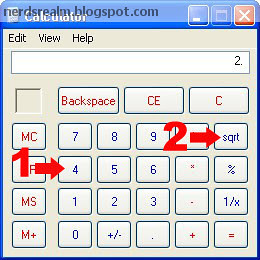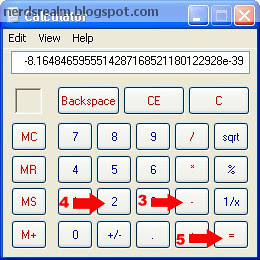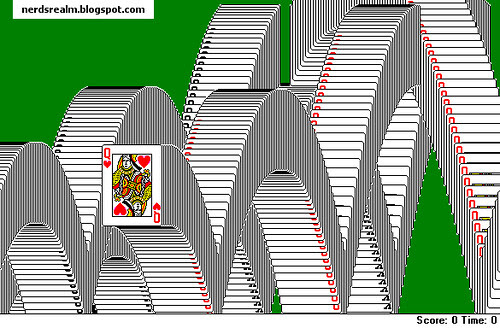# 2 Windows Easter Eggs You Have To See

"Easter Eggs (Definition) - A hidden message or feature in an application."

What is the square root of four?  The answer is two,

And what do you get if you subtract two from two? Zero, right?

Now let’s do the above calculation using the Windows calculator. Type in 4, take the square root and from the result subtract 2. What do you get now?

Step 1: In calculator press 4
Step 2: Press the Sqrt button  and you will get 2 which is the right answer.Step 3: Press the - subtract button
Step 4: Press 2 (to subtract 2) and then
Step 5: Press = button.For what I know the operation returns different numbers in different versions of Windows, so it’s possibly a bug by design or an Easter Egg and not some weird functional error. What was your result?

Second Egg: Windows XP only.

Everyone has played the famous solitaire game.

All you have to do is open Solitaire and click the following key combination: [ALT] + [SHIFT] +When you click the above key combination, the game ends instantly and you see the cards jumping to the front in waves, as they do when a game is completed successfully.

Share your own windows bugs! Which one is your favourite?

1.The first easter egg is actually a number representation error. It can happen with any platform that uses Floating Point (The way non whole numbers are stored in a computer) number representation. The square root operation is also not entirely accurate if i remember correctly as it uses an iterative approximation method.
The representation of numbers has a fixed number of possible values. With floating point, there is almost always a very very small error in the representation. Thats why the double precision floating point datatype exists.
The square root operation likely returned (2 - 8.1648465955514287168521180122928e-39) instead of 2.0. It shows as 2 because of the way Microsoft coded the number display. However when you minus 2 from that number, it displays what remains which is -8.1648465955514287168521180122928e-39.
This occurs with other calculated square root - actual square root operations. For example sqrt(9) - 3 = 1.1546388020691628168216106791278e-37.
Computer Programming is something I do so I have to know these things.

2.3.4.